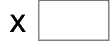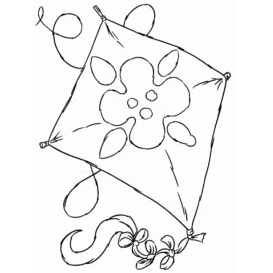14= 84   84= 19325= 435   14= 476   1= 100   75= 3675 1= 44   69= 6624   60= 5640   67= 5025